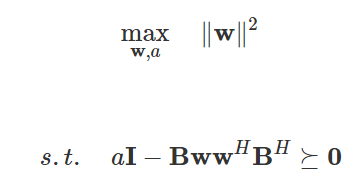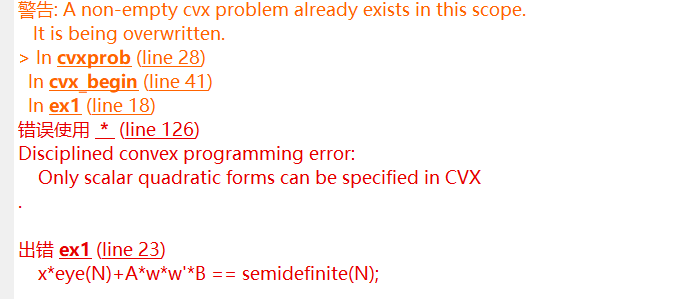# How to solve this problem using cvx?hello, i don’t know how to solve this problem using cvx,because there is as LMI as a constraint. Can you help me?

N = 10;
A = randn(10);
B = randn(10);
C = randn(10);
D = randn(10);
a = 0.5;
b= 5;

cvx_begin
variable w(N,1)
variable x
minimize (sum_square(w))
subject to
xeye(N)+Aw*w’*B == semidefinite(N);
cvx_endReformulate with Schur complement.
`[a*eye(10) B*w;w'*B' 1]==semidefinite(11)`

Your program is inconsistent with the image. I assume objective really is minimize (see below), otherwise this would be non-convex. And, as in the image, you need B and B’ in the semidefinite constraint, not A and B as in your program.

The image’s uses of H rather than T suggests `w` is intended to be complex. But I’ll let you fix up these details.

With the formulation as is, the solution is trivially all zeros for `w`. So perhaps the image is 'correct", and the objective is really to maximize. That would make the problem non-trivial, and also make it a non-convex nonlinear SDP for which CVX can’t be used.

thank you very much, i have got it.